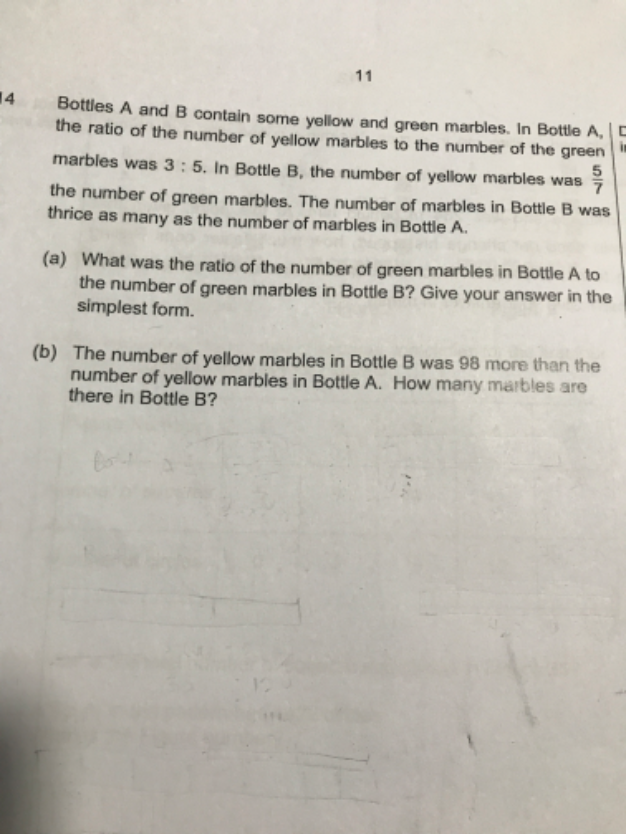# QuestionHi need assistance for this question. Thank you in advance

Bottle A (u)                                    Bottle B (3u)

Y  :    G       Total                             Y   :   G        Total

3   :    5         8                                  5     :   7         12

(a)   (5/8)u  /  (7/12)(3u)   =  (5/8) / (7/4) = 5/14

Hence  Bottle A  Green marbles : Bottle B Green marbles  =   5   : 14

(b) (5/12)(3u) – (3/8)u = 98

(5/4)u – (3/8) u = 98

10u – 3u = 98 x 8 = 784

7u = 784

u = 112

Hence Number of marbles in Bottle B = 3u = 3 x 112 = 336

0 Replies 2 Likes